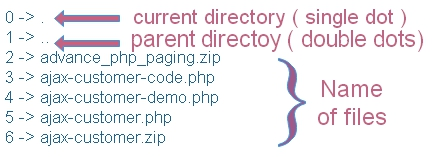# scandir(): List files and directories

``````\$ar=scandir('test_dir'); // Change this path
print_r(\$ar);``````
or
``````\$ar=scandir('C:\\dir_name\\abc\\'); // Change this path to directory
foreach (\$ar as \$key => \$val) {
echo "\$key -> \$val <br>";
}``````This function returns an array. Here is the syntax.
``Scandir('directory_sting',sort_order,'context');``
`Directy_sting`:
directory name with path as string
`Sort_order`: (Optional)
The listing order is ascending by default ( alphabetical ). Can by changed by SCANDIR_SORT_DESCENDING and Random by SCANDIR_SORT_NONE

Constants: Use 0 for ascending order and 1 for descending order.
`Context`:
(Optional)
From the Context parameter listing
As scandir output is an array, we can apply all array function to this output. Listing all files and directory of current folder
``````\$ar=scandir('.');
print_r(\$ar);``````
Listing all files and directory of up folder
``````\$ar=scandir('..');
print_r(\$ar);``````
We can develop a small script which will first list file and directory of current directory. All listed directory will have hyperlink to list files and directory present inside that on click. We have used is_dir function to check if the output string is a directory or not.
``````\$var=\$_GET['var'];
if(strlen(\$var)==0){
\$var='.';
}
\$ar=scandir(\$var);
while (list (\$key, \$val) = each (\$ar)) {

if(is_dir(\$val)){ // True if directory
echo "<a href=scandir.php?var=\$val>\$val</a><br>";
}else {
echo "\$val<br>";
}

}``````

## Travelling to different subdirectory and up directory structure

Above script we will modify to provide option where users can click folders to visit those folders. They can move upward ( towards root ) or can move downward by travelling to different levels deep inside the folder structure. Here is the code to do that.
``````<?php
@\$var=\$_GET['var'];
\$path = '';
if(strlen(\$var)==0){
\$path='';
}else{
\$path=\$var;
}
\$cwd=getcwd();
\$cwd=\$cwd.\$path;

chdir(\$cwd);

echo "<br>Current Working Directory : ".getcwd()."<br>";

\$ar=scandir(\$cwd);
while (list (\$key, \$val) = each (\$ar)) {
\$path2=\$path."".\$val;
if(is_dir(\$val)){
echo "<a href=dir-listing.php?var=\$path2>\$val</a><br>";
}else {
echo "\$val<br>";
}
}
?>``````

## Emptying folders by deleting all files

This is one of the way to empty the files in a folder to delete the folder. This script will keep on deleting files as you move on different level of folders.

Be warned that as you are going to delete the files not only of current folder but of all other folders you try to visit.

We kept the delete command commented for safety reasons and after understanding all the consequences you can un comment the line and use it at your own risk.
``````<?php
@\$var=\$_GET['var'];
\$path = '';
if(strlen(\$var)==0){
\$path='';
}else{
\$path=\$var;
}
\$cwd=getcwd();
\$cwd=\$cwd.\$path;

chdir(\$cwd);

echo "<br>Current Working Directory : ".getcwd()."<br>";

\$ar=scandir(\$cwd);
while (list (\$key, \$val) = each (\$ar)) {
\$path2=\$path."".\$val;
if(is_dir(\$val)){
echo "<a href=dir-listing.php?var=\$path2>\$val</a><br>";
}else {
if(\$val<>'dir-listing.php'){
// Uncommenting below line will delete your files of each folder you list ////
}
echo "\$val<br>";
}
}
?>``````

Subscribe to our YouTube Channel here

## Subscribe

* indicates required
Subscribe to plus2netplus2net.com